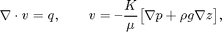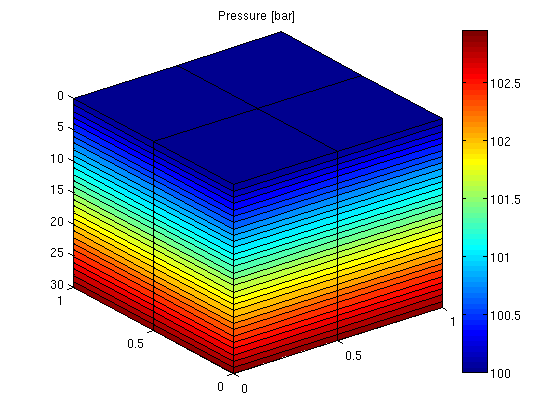#Gravity Column

In this example, we introduce a simple pressure solver and use it to solve the single-phase pressure equationwithin the domain [0,1]x[0,1]x[0,30] using a Cartesian grid with homogeneous isotropic permeability of 100 mD. The fluid has density 1000 kg/m^3 and viscosity 1 cP and the pressure is 100 bar at the top of the structure.

The purpose of the example is to show the basic steps for setting up, solving, and visualizing a flow problem. More details on the grid structure, the structure used to hold the solutions, and so on, are given in the basic flow-solver tutorial.

## Define the model

To set up a model, we need: a grid, rock properties (permeability), a fluid object with density and viscosity, and boundary conditions.

gravity reset on
G          = cartGrid([2, 2, 30], [1, 1, 30]);
G          = computeGeometry(G);
rock.perm  = repmat(100*milli*darcy(), [G.cells.num, 1]);
fluid      = initSingleFluid('mu' ,    1*centi*poise, ...
'rho', 1000*kilogram/meter^3);
bc  = pside([], G, 'TOP', 100.*barsa());


## Assemble and solve the linear system

To solve the flow problem, we use the standard two-point flux-approximation method (TPFA), which for a Cartesian grid is the same as a classical seven-point finite-difference scheme for Poisson's equation. This is done in two steps: first we compute the transmissibilities and then we assemble and solve the corresponding discrete system.

T   = computeTrans(G, rock);
sol = incompTPFA(initResSol(G, 0.0), G, T, fluid, 'bc', bc);


## Plot the face pressures

newplot;
plotFaces(G, 1:G.faces.num, convertTo(sol.facePressure, barsa()));
set(gca, 'ZDir', 'reverse'), title('Pressure [bar]')
view(3), colorbarPublished March 4, 2011